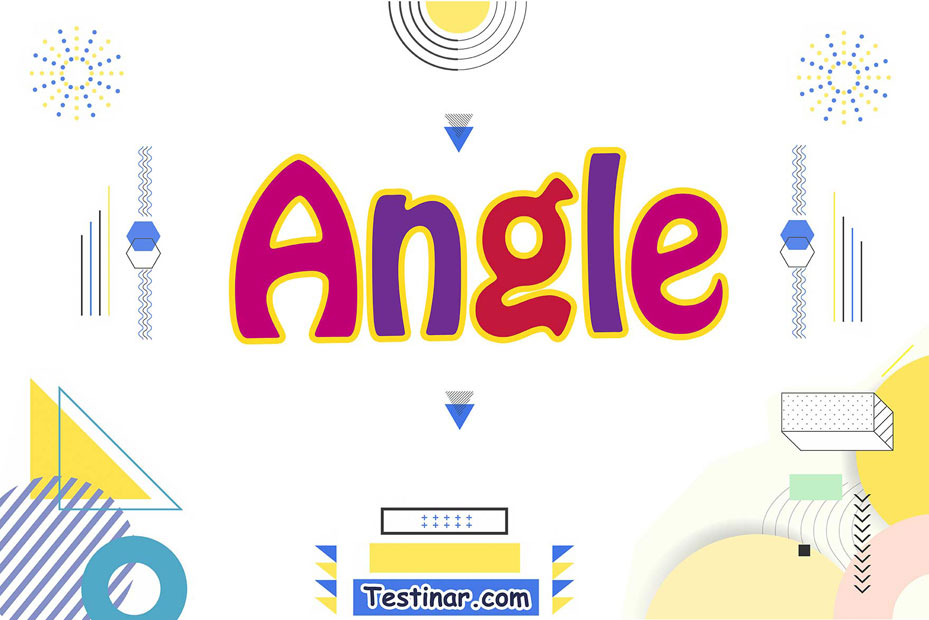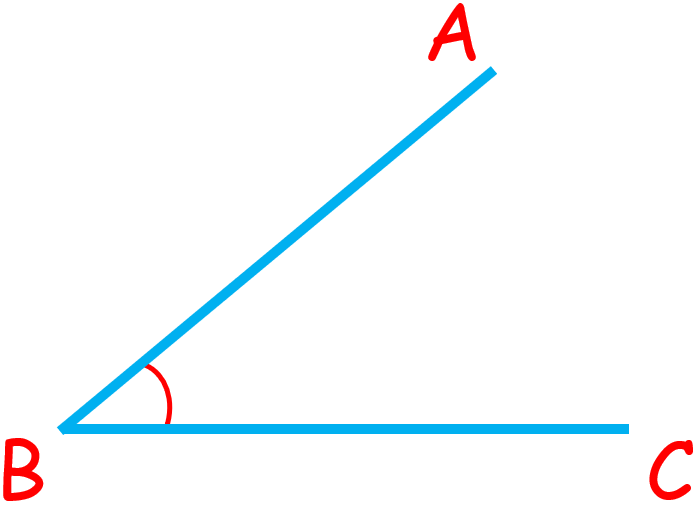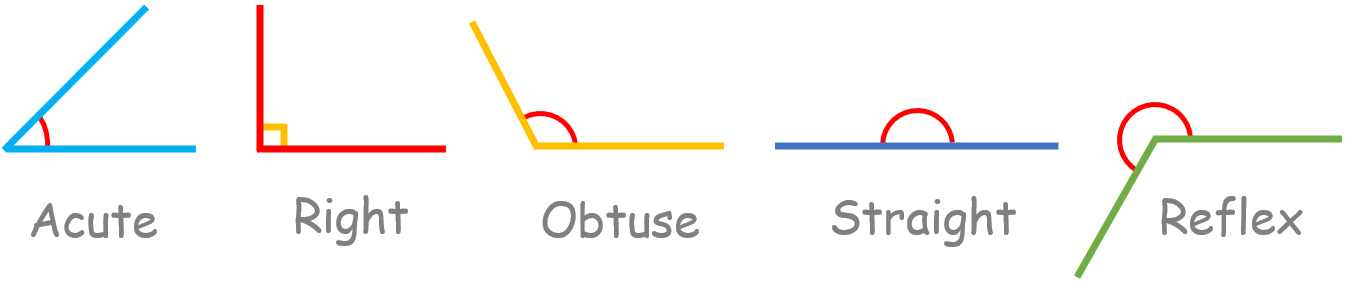## What is an angle?

### Definition of Angles

An angle is a type of geometric shape that is made by joining the ends of two rays together. The angle can also be shown by the three letters of the shape that makes the angle. The angle is in the middle letter (i.e.its vertex). Most of the time, Greek letters like $$β \ , \ α \ , \ φ$$, etc. are used to show angles.### Different Types of Angles

In Geometry, there are mostly six different kinds of angles. All of the angles and their properties are named as follows:

• An acute angle is between $$0°$$ and $$90°$$.
• A right angle is an angle that is exactly $$90$$ degrees.
• The obtuse angle is between $$90°$$ and $$180°$$.
• A straight angle is an angle that is exactly $$180$$ degrees.
• The angle that is greater than $$180$$ degrees and less than $$360$$ degrees is called the reflex angle.### Degree of an Angle

When the rotation from the starting side to the end side is $$\frac{1}{360}$$ of the full rotation, the angle is said to be $$1°$$.

### Exercises for Angles

1)2)3)4)5)6)7)8)9)10)1) $$\color{red}{144^o }$$2) $$\color{red}{60^o }$$3) $$\color{red}{102^o }$$4) $$\color{red}{96^o }$$5) $$\color{red}{54^o }$$6) $$\color{red}{138^o }$$7) $$\color{red}{48^o }$$8) $$\color{red}{90^o }$$9) $$\color{red}{132^o }$$10) $$\color{red}{42^o }$$## Angles Practice Quiz

### Prepare for the ACT Math Test in 7 Days

$14.99$12.99

### PSAT Math Practice Workbook

$25.99$14.99

### The Most Comprehensive TASC Math Preparation Bundle

$79.99$36.99

### AFOQT Math Full Study Guide

$25.99$13.99